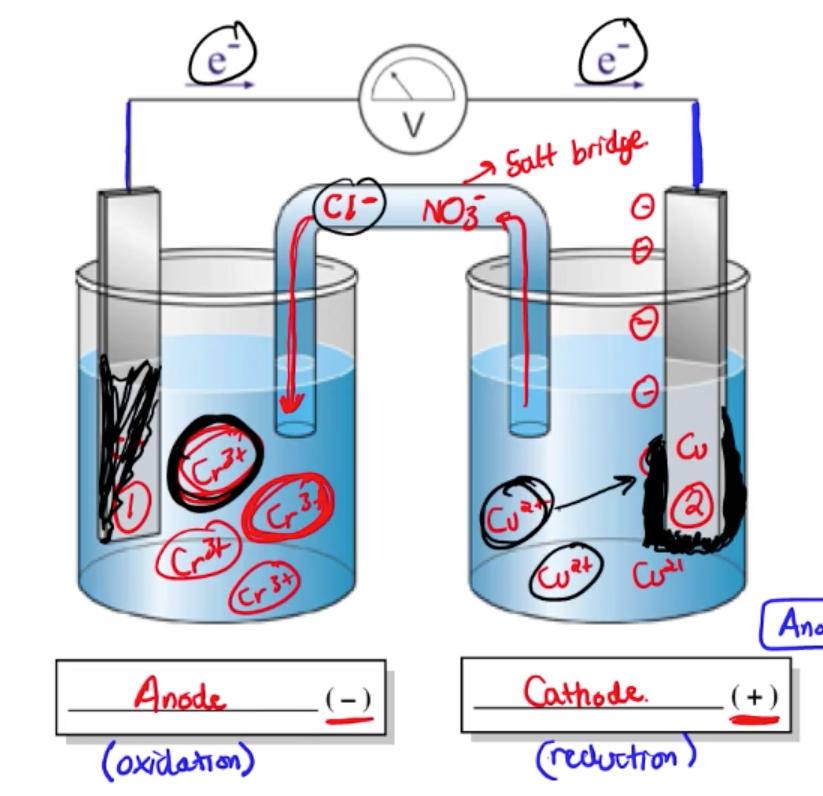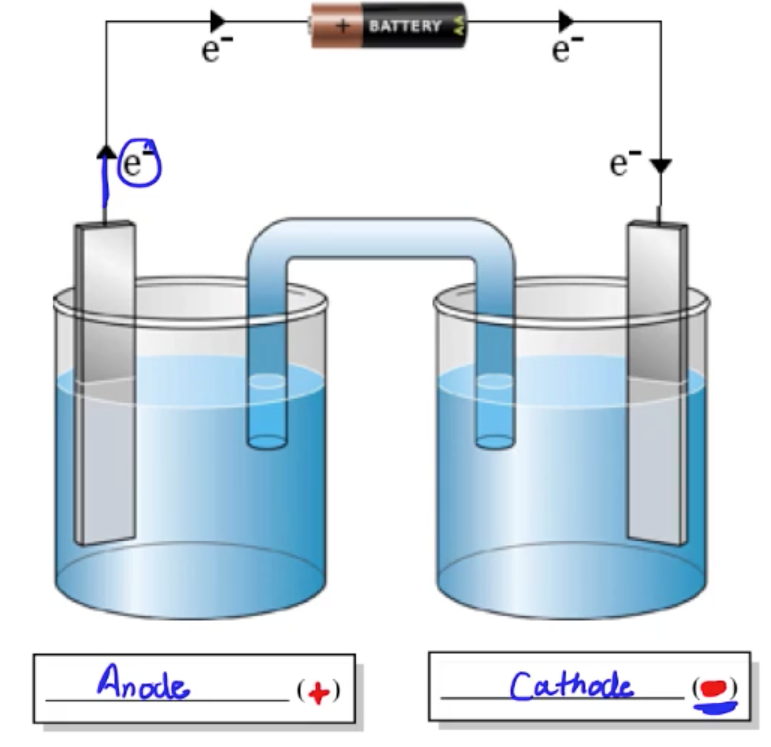Clutch Prep is now a part of Pearson
Ch.13 - Fundamentals of ElectrochemistryWorksheetSee all chapters

# Electrochemical Cells

See all sections
Sections
Basic Concepts
Electrochemical Cells
Standard Potentials
Nernst Equation
Standard Cell Potential & the Equilibrium Constant
###### Galvanic or Voltaic Cell

Concept #1: Galvanic or Voltaic cells represent spontaneous electrochemical cells that produce or discharge electricity.###### Electrolytic Cell

Concept #2: Electrolytic Cells represent nonspontaneous electrochemical cells that consume electricity.###### Line Notation

Concept #3: Line notation is a quick, simple method to describe an electrochemical cell without having to draw it out in detail.

Example #1: Write the half reactions as well as the overall net ionic equation for the following line notation:

Cu | Cu2+ (aq, 0.0050 M) || Ag+(aq, 0.50 M) | Ag

Example #2: Sketch the galvanic cell and determine the cell notation for the following redox reaction:

2 H+ (aq) + Fe (s) ⇌ H2 (g) + Fe2+ (aq)

Practice: Sketch the galvanic cell and determine the line notation for the following redox reaction:

Ni2+ (aq) + Mg (s) ⇌ Ni (s) + Mg 2+ (aq)